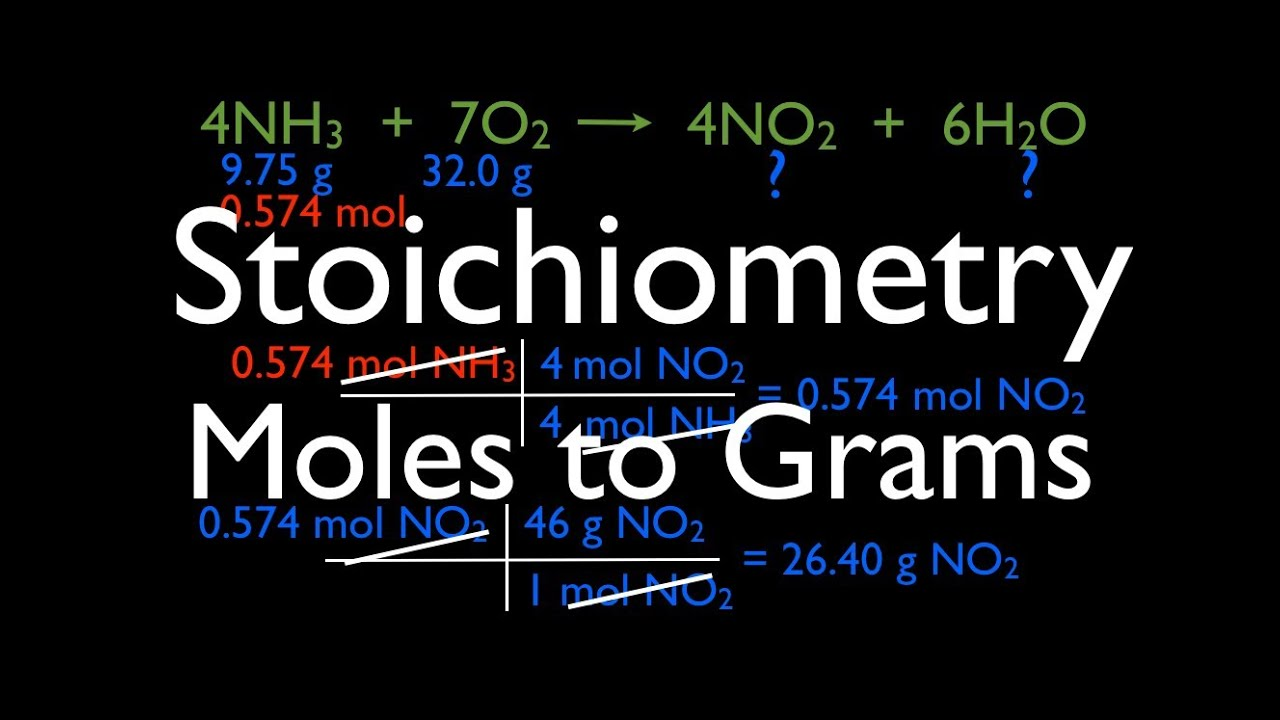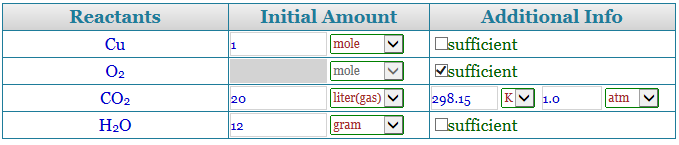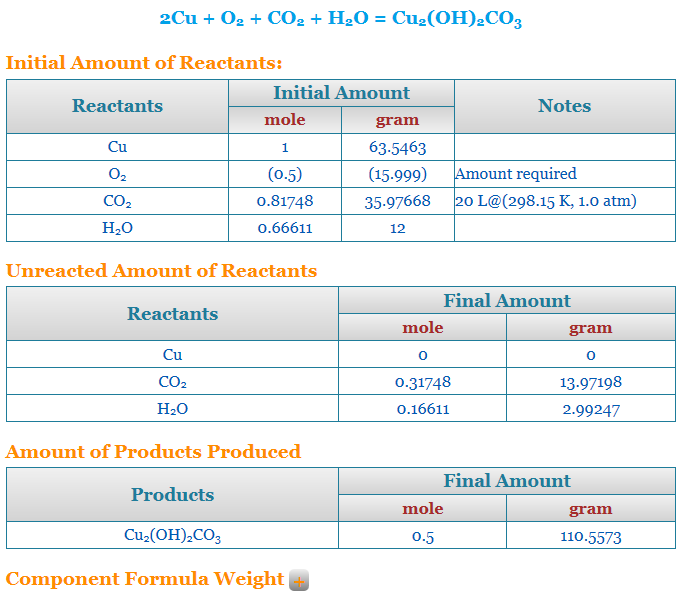# What is a stoichiometry calculator and how should you use it?

So, before we go into the details of what stoichiometry is or how we apply it in our lives, let us make a few basic things clear. Therefore, to begin with, we all must understand that intricate scientific calculations do not take place with our standard measurement units or SI units- be it physics or chemistry. Especially in a chemistry lab, you probably cannot ever have access to kilos, or even grams of a reactant or product. A little amount of any reactant can have very far-reaching outcomes. Therefore, to measure reactants, products, catalysts, and everything else, chemists designed a new measurement process that would serve the interest of chemists alone. Therefore, this is what we call stoichiometry. So, we will see how a stoichiometry calculator works or what is the function of a stoichiometry calculator. However, before that, we will go through its scientific definition and purpose.Hence, in Chemistry, stoichiometry is defined as the relationship between the relative quantities of substances- either reactant taking part in a reaction or products forming a compound. Moreover, it is generally a ratio of whole integers. So, this is what a stoichiometry calculator helps us attain. Moreover, there can be different kinds of conversion in chemistry. A stoichiometry calculator helps to sort them all. Therefore, you might want to convert moles to grams or vice-versa, grams to atoms or vice-versa, and so on.

Moreover, you would need to know what is molar mass, what is gram atomic mass, how to convert each, how to find the number of moles from a given sample of an element or a compound, and so on. Therefore, in the ensuing sections, we will see some of them and also how you can input such data into a stoichiometry calculator.

## Stoichiometry calculator moles to gram

So, before we go into the details of converting moles to grams and how a stoichiometry calculator works there, let us go through the individual definitions once. We all know that gram is a standard unit of measurement for the mass or weight of a substance. So, 1 kg = 1000 grams. However, we need to see in some detail what mole or molar mass is.

## Meaning of a mole

Therefore, 1 mole is the Avogadro number of some chemical units in chemistry. However, this unit can be different. It might be atoms, molecules, ions, or anything. The reason why scientists use the mole is that it makes intricate calculations easier. So, it becomes some kind of common ground between atoms, molecules, or ions- all of which are plenty in quantity. Hence, they also help in converting grams to atoms. So, in simple words, it is exactly 6.02214076×10^23, or the Avogadro number of any elementary entities or particles.## What do you mean by molar mass?

Therefore, after a mole, the question that definitely arises is what is molar mass. Moreover, how is it different from normal mass? However, chemists define molar mass as a ratio. The ratio is between the total mass of a substance and the number of moles that are present in the sample that we currently have. Moreover, the unit of molar mass is kg per mole. But we already know the value of 1 mole. So, we can easily find the mass in kgs and hence in gms.Therefore to convert moles to grams without using a stoichiometry calculator, we need to follow a few steps. Moreover, as per the given quantity, the moles and grams will differ, but the molecular mass will always remain the same.

So, at first, we have to find out the molecular mass if it is a compound and the atomic mass if it is an element. Then, you have to multiply this by the number of moles that you already know. So, this will give you the total amount of the substance present in grams.

Moreover, this is exactly the process that a stoichiometry calculator uses.

## Stoichiometry calculator mass to mass

So, this might seem a little confusing. However, thinking about it properly, mass to mass conversions are all that a stoichiometry calculator can do. So, the entire branch of stoichiometry concerns mass, but in different forms. You might need to calculate the mass lost in the process of a reaction, how much product turned up from how much reactant or how much react changed into energy, and similar stuff. However, in all cases, you have mass on both sides. You do not have room for much other quantity. But what you do is compare or convert them from one unit to another as per the suitability of the reaction or the question that you have at hand.

Moreover, remember that the unit of mass might also differ. That is the very purpose of a stoichiometry calculator. For example, let us consider moles. When you say moles, you know it is about the mass, but in reality, it is a ratio. It is a number of particles that exist in the sample. Hence, it has no ratio. However, that hardly matters. Similarly, the units of atomic and molar mass differ. The job of the stoichiometry calculator is to place the right unit in the right situation by making proper conversions. However, finally, it is your job to understand what is the conversion that you need to undertake after looking at a question.## Stoichiometry calculator with grams

So, grams are a very basic part of any stoichiometry calculator or calculation. In most cases, this is the unit we use to measure the total mass of the sample. Let us take an example of converting grams to atoms to help you understand the process.

So, atomic mass is the mass of an atom. Moreover, its unit is an atomic mass unit. So, the formula is-

1 amu = 1.661 x 10^-27 kg.

Therefore, 1 amu = 1.661 x 10^-27 x 10^3 gms

So, to convert grams to atoms-

1.661 x 10^-24 gms = 1 amu.

So, if you have any calculation involving amu where you need to get the value in gms, you can just multiply the value with 1.661 x 10^-24.

Now, there are a few things that we need to take note of-

1 Da or 1 amu is the atomic mass constant which we are basically multiplying to find the value in kgs or grams.

So, 1 amu = Molar mass constant/ Avogadro’s constant.

However, the molar mass constant that we use in converting grams to atoms is the molar mass of the C-12 isotope. So, this is the total mass of C-12 divided by the number of moles- 12. Moreover, Avogadro’s constant is 6.02214076 × 1023. So, it is the number of units that you can find in 1 mole of any substance.

Therefore, 1 amu = M (C-12)/ (12 x Avogadro’s number) = 1.661 x 10^-27 kg. So, in this process of converting grams to atoms, M is the molar mass of C-12. So, we must know what a mole or molar mass is.## Stoichiometry calculator free

The internet makes everything very easy. Therefore, it is the same with a stoichiometry calculator. However, it is very helpful for budding students. In case, the process is still not clear, you might want to go through the stoichiometry calculator. This will help you understand how to calculate a sum and show you the results stepwise. Moreover, we humans tend to make a lot of mistakes whenever we do any calculations manually. In calculations like these, where we handle very intricate quantities, a little mistake can have a far-reaching impact that is not desirable. So, a stoichiometry calculator helps one on that ground as well. Using it makes the process much safer than manual calculation.

Moreover, it might so happen that you know the process and all but the answer still does not match. So, in that case, just run through the stoichiometry calculator. A step-by-step analysis will show you if you had made any mistake and where you made it. So, this will help you to rectify the next time. Therefore, a stoichiometry calculator is not explicitly for beginners. Sure beginners might need it more than anybody else. However, it is for everyone who is in this field and has to do regular calculations. It is always better to make a run no matter how good you are at it to ensure that the calculation is absolutely correct.## Stoichiometry calculator with steps

So, now let us see how to use a stoichiometry calculator with steps from scratch. You will of course find many comprehensive calculators online but here we will concentrate on only one.

## Step 1 to use a stoichiometry calculator

Therefore, we will use the stoichiometry calculator from Thermobook here. So, click on Thermobook. This will land you directly on the page containing the calculator. Now, once you reach the page, you will find a number of sections. So you will find the calculator at the top of the page. However, under it, you will also find a section containing examples only. These will help you understand the working of a stoichiometry calculator even when you do not have a question at hand. So, this is exactly how you will have to solve a sum.

Now, in the calculator, you have two boxes that look like a search engine. So, in one, you have to enter the equation and in the other the type of stoichiometric calculation that you want to undertake.

## Step 2 to use a stoichiometry calculator

So now you enter the equation under the “input the equation” box of the stoichiometry calculator. It is not necessary to balance the equation. If you can- that is fair enough. Let us say, the equation here is H2 + 02 -> H20. Now go to the next option that says “calculation type”. So click the “select one” option on the box beside. Once you click it, you will have two options- “reactant amount given” and “product amount given”. So, you will have to select one amongst these two depending on whether you have the quantity of products or reactants or what you need to find out. Let us say, in this case we know the quantity of the reactants and need to know the quantity of the products.## Step 3 to use a stoichiometry calculator

Now, a table will appear containing the name of the reactants and the parallel number of moles that are present. In case you have the quantity in grams, change it to moles simply by dividing it with the molecular mass of the reactants. So, let us say we have 4 moles of hydrogen and 2 moles of oxygen. Enter the values. There is also a place for some additional info that you can fill up depending on the question at hand.

## Step 4 to use a stoichiometry calculator

Now tap the blue button at the right-hand side bottom that reads “calculate”. It will take a few seconds to complete the calculation. So, you will find the number of moles that are produced by the product. Moreover, you will also find it in the gram format. In this case, 4 moles of water are produced. Moreover, there is also an option to find out the compound formula weight. In this case, the value is 18.015 for water, 2.016 for hydrogen, and 31.998 for oxygen.## Stoichiometry calculator limiting reactant

So this stoichiometry calculator finds out the limiting reactant for an equation. In this case, we are using the free stoichiometry calculator from Chemical Aid. Therefore, in the beginning, you have to enter the equation whose limiting reactant you have to find. However, it is not necessary for the equation to be properly balanced. Let us say in this case the equation is NaOH + HCl = NaCl + H20.

So now, the stoichiometry calculator will itself provide the molar mass of all the reactants and the products. However, you have to enter the weight in grams as well as the moles of all the products and reactants. So after you do this, you can either ask the calculator to balance the reaction or calculate reaction stoichiometry. Now, since the equation will be balanced, and your values entered are arbitrary there will be one or more reagents that are not in the proper quantity with respect to the rest of the reactants and products to balance the equation. Therefore, this one becomes the limiting reactant. So, your stoichiometry calculator will give the name of the reagent as well as what has to be done to fix it.

## Stoichiometry calculator FAQs

### What does a stoichiometry calculator allow us to do?

Ans. So, a stoichiometry calculator allows us to find out and compare various kinds of quantitative data. It also helps us to convert various mass forms into one another. Moreover, there are also stoichiometry calculators that help you find out things like enthalpy and limiting reagents. However, they are a little more advanced.

### What are the concepts in stoichiometry?

Ans. So, stoichiometry basically calculates or predicts the quantity of either reactants or products. Therefore, you must know the molar mass, conversion to moles, role of limiting reagent, and definitely how to balance even complex equations. These are very important for any stoichiometric calculation.

### How does stoichiometry support the law of conservation of mass?

Ans. Well, stoichiometry is based upon the law of conservation of mass itself. The law of conservation of mass says mass can neither be created nor destroyed. So, it can only be transformed from one form to another, or into energy. Moreover, in a balanced chemical reaction, the total amount of reactants will always be the same as the total number of products. Therefore, the mass remains constant, following the law. Moreover, this is why balancing becomes so important in stoichiometry.

### What is the first thing you do while solving a stoichiometry problem?

Ans. Well, if you are not using a stoichiometry calculator, the first job is to balance a chemical equation properly. If this is not correct, your entire sum will go wrong and become meaningless. Moreover, the formulas of the compound also need to be correctly written. However, if you are using a calculator, you do not need to worry since it will do it for you.

### Does b2h6 react with water?

Ans. Yes, it does. Moreover, diborane reacts very violently with water. The final products are hydrogen and boric acid. The equation for this is- B2H6 + 6 H2O → 2 B(OH)3 + 6 H2 (ΔHr = −466 kJ/mol = −16.82 kJ/g)